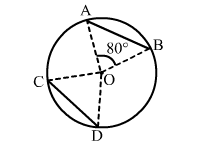# AB and CD are two equal chords of a circle with centre O such that ∠AOB = 80°, then ∠COD = ?

Question:

AB and CD are two equal chords of a circle with centre O such that AOB = 80°, then ∠COD = ?
(a) 100°
(b) 80°
(c) 120°
(d) 40°Solution:

(b) 80°
Given: AB = CD
We know that equal chords of a circle subtend equal angles at the centre.
∴ ∠COD = ∠AOB = 80°